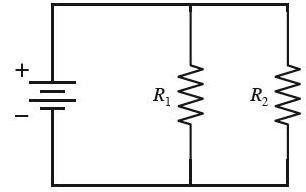# Problem: The circuit below has two resistors, with R1 &gt; R2. Which of the two resistors dissipates the larger amount of power?

###### FREE Expert Solution

R1 is greater than R2.

The voltage across both resistors is the same — parallel resistors.

Electrical power,

$\overline{){\mathbf{P}}{\mathbf{=}}\frac{{\mathbf{V}}^{\mathbf{2}}}{\mathbf{R}}}$###### Problem DetailsThe circuit below has two resistors, with R1 > R2. Which of the two resistors dissipates the larger amount of power?